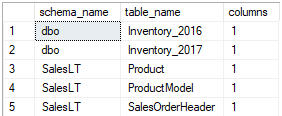## Find tables with large object (LOB) data type columns in Azure SQL Database

Article for:

Large objects in Azure SQL Database are columns with the following data types: varchar(max), nvarchar(max), text, ntext, image, varbinary(max), and xml.

The query below lists all tables that contain columns with LOB data types.

## Query

``````select t.table_schema as schema_name,
t.table_name,
count(*) as columns
from information_schema.columns as c
inner join INFORMATION_SCHEMA.tables as t
on c.TABLE_SCHEMA = t.TABLE_SCHEMA
and c.TABLE_NAME = t.TABLE_NAME
where t.TABLE_TYPE = 'BASE TABLE'
and ((c.data_type in ('VARCHAR', 'NVARCHAR')
and c.character_maximum_length = -1)
or data_type in ('TEXT', 'NTEXT', 'IMAGE', 'VARBINARY', 'XML', 'FILESTREAM'))
group by t.table_schema,
t.table_name
order by t.table_schema,
t.table_name
``````

## Columns

• schema_name - name of the schema
• table_name - name of the table
• columns - number of LOB columns in a table

## Rows

• One row: represents one table
• Scope of rows: all tables containing columns with LOB data types in the current database
• Ordered by: schema name, table name

## Sample results

List of tables with LOB columns in the AdventureWorksLT database: